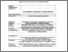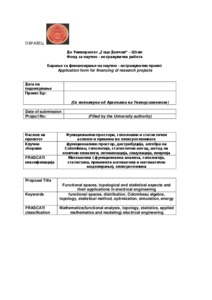# Functional Spaces, Topological and Statistical Aspects and Their Applications in Electrical Engineering

Atanasova-Pacemska, Tatjana and Jolevska-Tuneska, Biljana and Cingoski, Vlatko and Lazarova, Limonka and Zlatanovska, Biljana and Miteva, Marija and Kostadinova, Slavica and Vasileva, Liljana and Gelova, Elena (2013) Functional Spaces, Topological and Statistical Aspects and Their Applications in Electrical Engineering. [Project] (In Press)Preview Text Konecen formular za aplikacija-proekt.pdf Download (434kB) | Preview

## Abstract

As it is well known, standard product can not be properly defined in the Schwartz distribution space and this is so called Schwartz impossibility result. This problem is overcome by Colombeau. In the framework of the Colombeau algebra, in order to multiply two distributions we first replace them by families of smooth functions and which converge in the sense of distributions toward f and g, respectively. Then, we can define product between the distributions f and g as the family .
Still, from a viewpoint of applications, this approach is useful only if has a "distributional shadow", i.e. if it converges in the sense of distributions to a distribution . On the other hand, for instance, it is well known that it is not possible to find a weak approximation of the distribution so that converges in (up to a multiplication constant).
So, in the framework of this project we will introduce the "new distribution space", a distribution space on discontinuous test functions which will be describe topologically. Also we will introduce a type of weak convergence analogically with convergence in the Colombeau algebra in order to get the convergence of . All of this will provide the conclusion .
Moreover, such result can be physically relevant. The results will be used in electrical engineering processes, social processes,…Some statistical and optimization methods will be used.
A part of the research would be concentrated on utilization of the first order integral and differential equation for simulation of dynamics of rotating electrical machines. An original mathematical model would be investigated and developed that, as close as possible, could describe the dynamics of the simulated object using a system of n-equations with n-unknown variables. As a result obtaining of dynamic response curves for various electromagnetic and electromechanical variables is envisaged. Obtained data would be used later on for analysis of the operational characteristics of the rotating machines, such as analysis of currents, speed and torque.

Item Type: Project Engineering and Technology > Electrical engineering, electronic engineering, information engineeringNatural sciences > Matematics Faculty of Electrical Engineering Tatjana A. Pacemska 12 Sep 2013 12:14 09 Mar 2015 10:11 https://eprints.ugd.edu.mk/id/eprint/7224View Item